# Test: Patterns - 2

## 10 Questions MCQ Test Mathematics for Class 5 | Test: Patterns - 2

Description
Attempt Test: Patterns - 2 | 10 questions in 10 minutes | Mock test for Class 5 preparation | Free important questions MCQ to study Mathematics for Class 5 for Class 5 Exam | Download free PDF with solutions
QUESTION: 1

### What is the missing number in the series given below?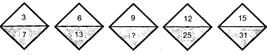Solution:

The pattern is as follows: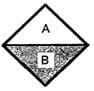B = A × 2 + 1 Hence, number in lower part of third pattern is: 9 × 2 + 1 = 19

QUESTION: 2

### If the pattern continues, what letter is in the 99th position? C B A A B C C B A A B C 1st....................10th

Solution:

The pattern repeats after 6 letters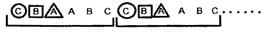So, now position of C B A 97th 98th  99th

QUESTION: 3

### How many shaded squares will be there in pattern 20?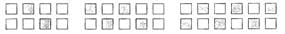Solution:

Number of shaded squares in pattern 1=2 Number of shaded squares in pattern 2=4 Number of shaded squares in pattern 3=6 So, Pattern followed is (1×2),(2×2),(3×2),....i.e., 2,4,6,... Hence, Number of shaded squares in pattern 20 =    20×2=40.

QUESTION: 4

Which figure will replace the question mark in the figure pattern below?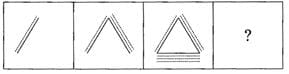Solution:

Each figure repeats itself after 2 figure.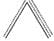QUESTION: 5

Which number will replace (?) in the number series below?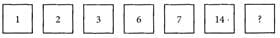Solution:

The pattern is as follows: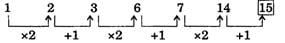QUESTION: 6

Which is the missing number in number matrix?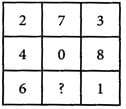Solution:

Rule followed column wise = Sum of the numbers in each column is = 12.

QUESTION: 7

What is the sum of the numbers in pattern 4?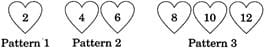Solution:

Numbers in pattern 4=14, 16, 18, 20 So, the total sum =14+16+18+20=68

QUESTION: 8

Write the number that continues each sequence in the most sensible way.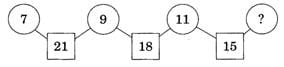Solution: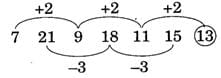QUESTION: 9

What is the missing number in the given number pattern?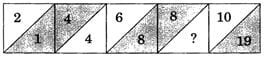Solution:

The pattern is as follows:
(i)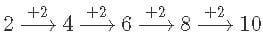(ii)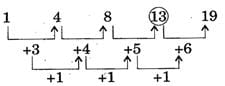QUESTION: 10

Find the missing term in the given pattern.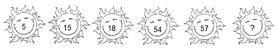Solution:

The pattern is as follows: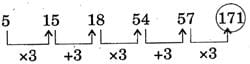Use Code STAYHOME200 and get INR 200 additional OFF Use Coupon Code